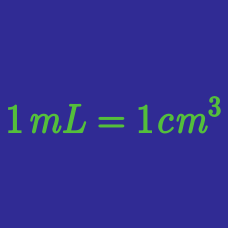Classical Mechanics

SI Divisor Prefixes

A capacitor is specified as having a value of $0.00{9}{9} \,\mu\text{F}.$ What is this value in picofarads?

An inductor has a value of $0.2 9 \text{ mH}.$ What is this in microhenrys?

Find the expression for a particular electric current $3.7 \times 10 ^{-6} \text{ A}$ by using the appropriate prefix of SI units.

Which of the following prefixes represents $1 \text{ micro } \times 1 \text{ nano }$ of a given unit?

Which of the following symbol of prefixes represents $10^{-6}$ of a given unit?

×